Computational & Technology Resources
an online resource for computational,
engineering & technology publications
Civil-Comp Proceedings
ISSN 1759-3433
CCP: 75
PROCEEDINGS OF THE SIXTH INTERNATIONAL CONFERENCE ON COMPUTATIONAL STRUCTURES TECHNOLOGY
Edited by: B.H.V. Topping and Z. Bittnar
Paper 3

Area Integral of a Stress Function over a Beam Cross-Section

R. Vondrácek and Z. Bittnar

Department of Structural Mechanics, Faculty of Civil Engineering, Czech Technical University in Prague, Czech Republic

Full Bibliographic Reference for this paper
R. Vondrácek, Z. Bittnar, "Area Integral of a Stress Function over a Beam Cross-Section", in B.H.V. Topping, Z. Bittnar, (Editors), "Proceedings of the Sixth International Conference on Computational Structures Technology", Civil-Comp Press, Stirlingshire, UK, Paper 3, 2002. doi:10.4203/ccp.75.3
Keywords: stress function, stress integration, cross-section, Green's theorem, surface integral.

Summary
The aim of this contribution is to present an algorithmically simple and mathematically efficient method of integration of a stress function over the area of a cross-section. For this purpose we transform area integral over the area of the cross section into a path integral along the border of the cross-section polygon by means of the Green's Theorem (3.1).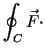d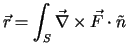d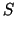(3.1)

Imagine a given plane of deformation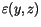over the cross-section and a given stress-strain function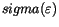describing material behavior. Our work shows that if we are able to derive analytical expressions for following primitive functions of the stress-strain function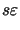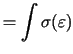d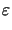(3.2)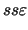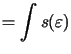d(3.3)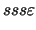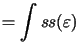d(3.4)

We can obtain exact analytical expressions for the cross-section internal forces. The constitutive stress-strain function are usually expressed as a continuous function by parts polynomial. Such functions can be easily integrated in a closed form and therefore we get (3.2), (3.3), (3.4) without any hard work.

For illustration of the process we present here a simple example. The concrete L shaped cross-section is described with an irregular polygon. The constitutive stress- strain function is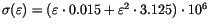MPa. From an affiliated analysis we obtain linearly distributed strain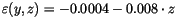. The task is to compute the corresponding internal forces quickly and accurately. In this example we limit us only to a bending moment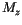and use derived integral expressions to evaluate the resultant.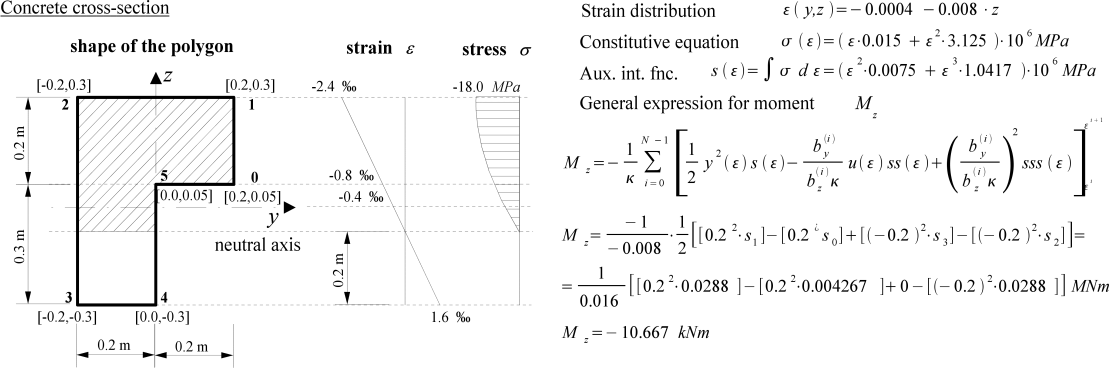The presented path integration of non-linearly distributed stress over the cross- section is a very powerful computational method. This method is extremely useful for the concrete reinforcement design and assessment, especially due to its algorithmic efficiency. The method enables us to operate with cross sections of different shapes and materials. The runtime of the integration is determined by the complexity of the defined cross section shape. This means that we can model any arbitrary shape of cross-section that can be approximated with a line polygon without any restrictions as for orthogonality or orientation. Nevertheless, if we work with a simple rectangular cross section loaded in the direction of its symmetry plane, we obtain as efficient expression of the integration of stress function as possible.

References
1
R. Vondrácek, "Numerical methods in nonlinear concrete design", Master's thesis, Czech Technical University in Prague 2001.
2
Z. Bittnar, J. Sejnoha, "Numerical methods in structural mechanics I-II", ES CVUT, Prague 1992

purchase the full-text of this paper (price £20)